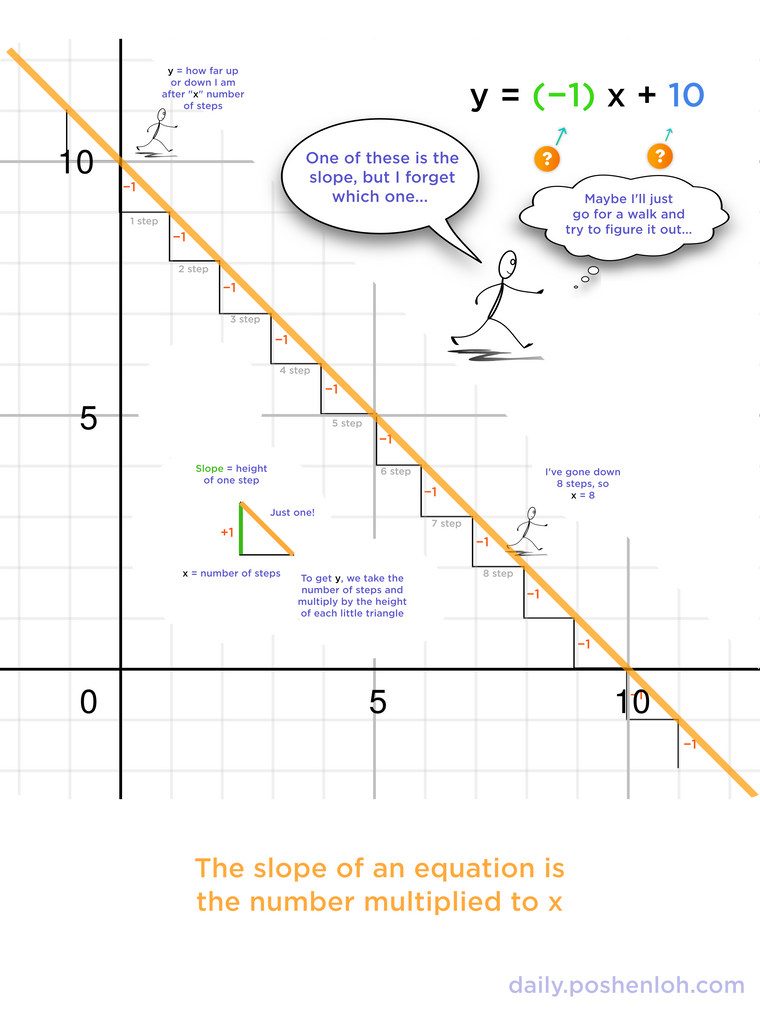# In the equation y = ax + b, which coefficient is the slope?

• When you learn the equation of a line for the first time, the concept of slope generally is rather quick to understand (it's how "steep" the line is), but it's easy to forget which coefficient is equal to the slope of the line.

\$\$ y = ax + b \$\$

Which is it??? The \(a\) or the \(b\) coefficient?

With a little bit of putting two-and-two together, you don't need to search in your textbook or ask Google. You can figure this out yourself!• I like to remember that an equation in the form of \(y = ax + b \) is called slope intercept form, so the first coefficient (a) is the slope, and the second coefficient (b) is the y-intercept.